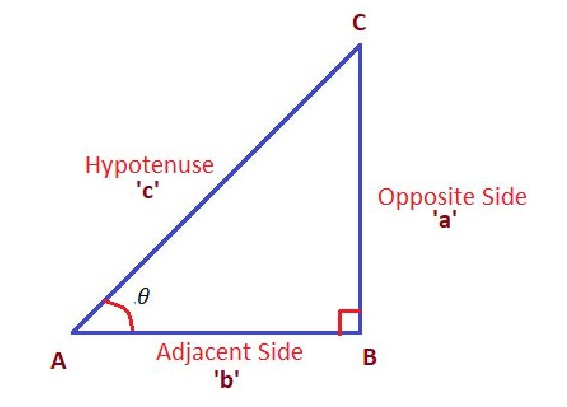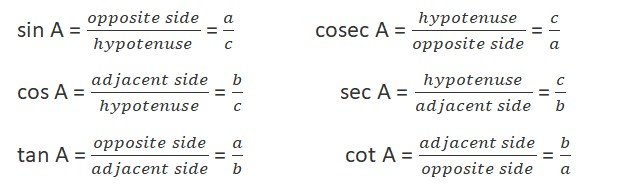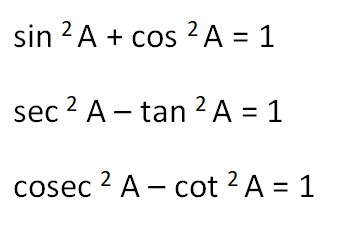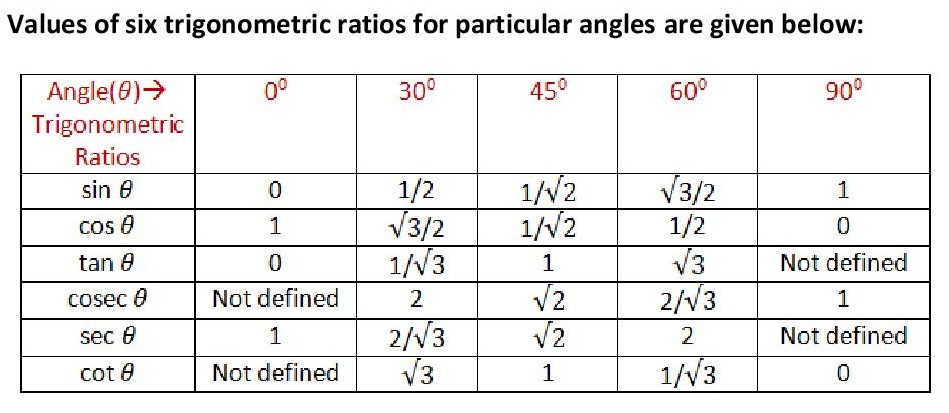Top

# GCSE Trigonometric

### INTRODUCTION

Trigonometry (derived from Greek words ‘trigonon’ means ‘triangle’ and ‘metric’ means ‘measure’) deals with unique relationships between angles and side lengths of triangles. It provides a systematic method for finding unknown sides and angles of the triangle. The properties of the right-angled triangle are studied here.

Trigonometric Ratios:

There are six trigonometric ratios which gives a relation between an angle of a right-angled triangle to ratios of any of the lengths of its sides.In the above right-angled triangle with vertices ABC,

• The side opposite to right angle is called Hypotenuse, which is the longest side (AC).
• The side opposite to angle is called as opposite side (BC).
• The side adjacent to angle is called as adjacent side (AB).

The ratios of different pairs of sides of the right angle are called trigonometric ratios of its acute angle with respect to angle A. The trigonometric ratios namely sine (sin), cosine (cos), tangent (tan), cosecant (cosec), secant (sec), and cotangent (cot) are defined asThe basic trigonometric ratios are sin, cos and tan while the rest three are the inverse of these basic functions.

Reciprocal Relations:Trigonometric Identities:

The trigonometric identities which specify the relation between these functions are given below:These identities are derived from the Pythagorean Theorem, which states that “In a right-angled triangle, square of the hypotenuse is equal to the sum of the squares of the other two sides”. This can be written as an equation relating the lengths of the sides a, b and c as:

Hypotenuse^2 = (Adjacent Side)^2 + (Opposite Side)^2

AC^2 = BC^2 + AB^2

c^2 = a^2 + b^2